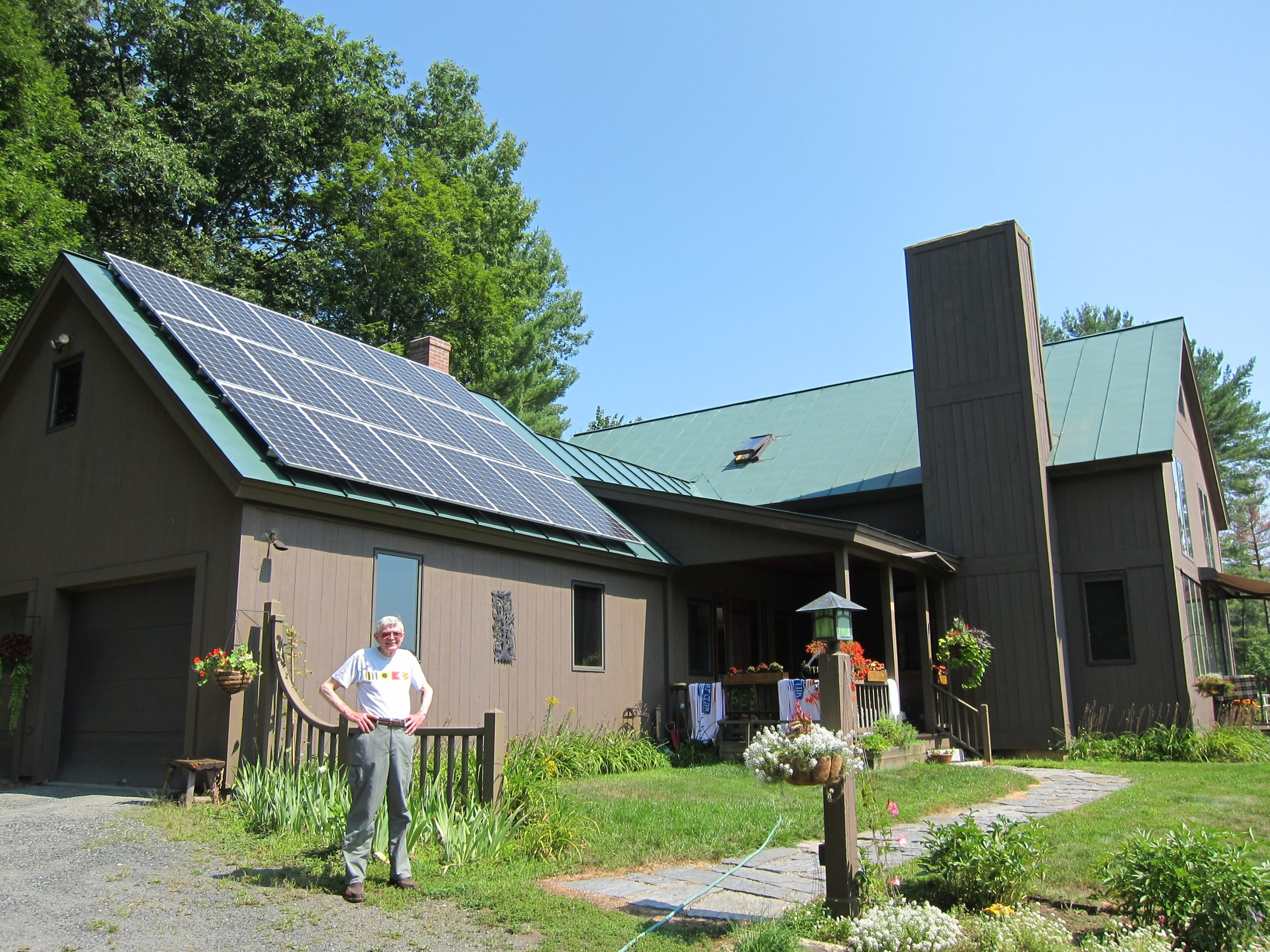# Calculate the electricity consumption of a house – MessHallThe electricity supply is a monthly expense of any home or business, where the user has to take care of this amount through the electricity bill and make a moderate consumption at the same time that it can be an intelligent economic benefit for him.### What is electricity consumption?

The power consumption is the amount of energy required for a given supply point for a period of time called billing period. This aspect is invoiced by the marketers by applying a price per kWh that determines the amount of money that the customer will have to pay.

### How to calculate electricity consumption?

There are several ways to calculate the electricity consumption of a home:

1.There are several ways to calculate the electricity consumption of a home

2.On the other hand, you can use an electricity consumption calculator , a tool that estimates the electricity consumption of a home through the appliances used and the number of people in the home. There are also simulators that allow you to calculate your consumption in money.

3.It can also be calculated through an electricity consumption meter , a device that is used to know at all times the use that is being made of energy. It can be very useful since knowing at all times the consumption that is made, you can reach save up to 15% on your electricity bill.

### What is the formula to calculate electricity consumption?

.What is the formula to calculate electricity consumption?

.Electric power

.Operating time

### What is the monthly kWh consumption in a house?

The consumption of a property is the part that raises the electricity bill the most, which is why it is convenient to make responsible consumption in the supply of electricity and thus reduce the amount to be paid every month. Two widely used ways to calculate monthly electricity consumption are the following:

1.Using an application to estimate electricity consumption is the fastest, free and most comfortable way. To do this, you only need basic data such as square meters, people who live together at home, connected appliances and spending habits.

2.The user can make the decision to measure the consumption by himself, adding one by one the cost of each of the electrical appliances connected per month and applying it in the aforementioned formula.

The following table shows the cost of electricity incurred in a home, taking as a reference the following list of connected electrical appliances and providing the total at the end of it. With this information, it is possible to

calculate the average electricity consumption in the home:

Electrical appliance        Load   use per day        Usage time per month  consumption per month

Heater      1.2kW   2 hours a day.    60 hours a month.           72kWh.

Fridge*                0.89kW 24 hours a day   720 hours per month.    320.4kWh.

Washing machine           0.33kW 1 hours a day.    30 hours a month.           9.9kWh.

Oven     0.79kW 5 hours a day.    150 hours per month.    118.5kWh.

Television           0.156kW              8 hours per day.               240 hours per month.    37.44kWh.

Lighting                0.24kW 6 hours a day.    180 hours a month.         43.2kWh.

Computer           0.01kW 6 hours a day     180 hours a month.         1.8kWh.

Microwave         1.2kW   3 hours a day.    90 hours a month.           108kWh.

Air conditioner 1.35kW 4 hours a day.    120 hours per month.    162kWh.

Total                                                     685.24kWh.

### What to do to reduce electricity consumption at home?

There are concepts within the electricity bill in which by modifying them we can save on costs:

1.Consumption: is the amount of energy that is used during the billing period. By controlling the consumption that is done monthly, it is possible to reduce the cost of the variable part that is applied to the electricity bill.

2.Power: is the amount of kW contracted. The lower the contracted power, the lower fixed costs will be paid on the electricity bill.

Further, There are also other electrical appliances considered low consumption, which contribute to energy savings in the home. They are very common to use, especially in winter, when electricity consumption multiplies. Here are some examples of them that you can start using in your home: